# Shadow of tree

Miro stands under a tree and watching its shadow and shadow of the tree. Miro is 180 cm tall and its shade is 1.5 m long. The shadow of the tree is three times as long as Miro's shadow. How tall is the tree in meters?

Result

h =  5.4 m

#### Solution:Leave us a comment of example and its solution (i.e. if it is still somewhat unclear...):

Showing 0 comments:Be the first to comment!#### To solve this example are needed these knowledge from mathematics:

Do you want to convert length units? See also our right triangle calculator. See also our trigonometric triangle calculator. Try conversion angle units angle degrees, minutes, seconds, radians, grads.

## Next similar examples:

1. Ruler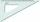How far from Peter stands 2m hight John? Petr is looking to John over ruler that keeps at arm's distant 60 cm from the eye and on the ruler John measured the height of 15 mm.
2. Plane IIA plane flew 50 km on a bearing 63degrees20 and the flew on a bearing 153degrees20 for 140km. Find the distance between the starting point and the ending point
3. Plan scaleAt what scale is drawn plan of the building, where one side of the building is 45 meters long is on the plan expressed by a straight line 12 mm long.
4. Scale of the mapDetermine the scale of the map if the actual distance between A and B is 720 km and distance on the map is 20 cm.
5. Feet to milesA student runs 2640 feet. If the student runs an additional 7920 feet, how many total miles does the student run?
6. Center traverseIt is true that the middle traverse bisects the triangle?
7. Degrees to radiansConvert magnitude of the angle α = 9°39'15" to radians:
8. Obtuse angleWhich obtuse angle is creating clocks at 17:00?
9. Mirror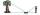How far must Paul place a mirror to see the top of the tower 12 m high? The height of Paul's eyes above the horizontal plane is 160 cm and Paul is from the tower distant 20 m.
10. SimilarityAre two right triangles similar to each other if the first one has a acute angle 70° and second one has acute angle 20°?
11. AnglesThe outer angle of the triangle ABC at the vertex A is 114°12'. The outer angle at the vertex B is 139°18'. What size is the internal angle at the vertex C?
12. Complementary angles 2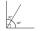Two complementary angles are (x+4) and (2x - 7) find the value of x
13. Acute angles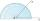Sizes of acute angles in the right-angled triangle are in the ratio 1: 3. What is size of the larger of them?
14. Ethernet cez ulicuKarol a Jozef sú vášniví hráči počítačových hier a býva v domoch, ktoré sú presne naproti sebe cez ulicu, takže si vidia navzájom do okien. Rozhodli sa, že si svoje počítače prepoja telefónnym káblom aby mohli hrať spoločne hry. Karol býva v prvom poschodí
15. ThomasThomas lives 400 meters away from Samko, Robo from Thomas also 400 m and Samko from Robo 500. Anton lives 300 meters away from Robo further as Samko. How far away lives Anton from Rob?
16. Type of triangle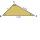How do I find the triangle type if the angle ratio is 2:3:7 ?
17. SimilarityABC is a triangle wherein a = 4 cm, b = 6 cm, c = 8 cm. Is it similar to the triangle DEF: d = 3 cm, e = 4.5 cm, f = 6 cm? If so, determine the ratio of similarity.